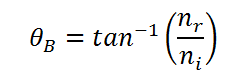# Calculating Polarizing / Brewster's angle

This CalcTown calculator calculates the Polarizing angle / Brewster's angle at which, the reflected light gets linearly polarized.

#### Result

degreeswhere

θB=Brewster's angle

ni=Refractive index of medium 1

nr=Refractive index of medium 2